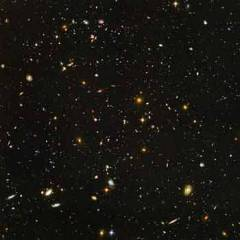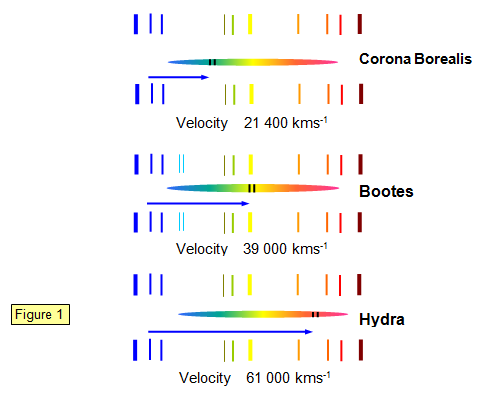# The speed of the galaxiesEarly in the twentieth century astronomers used the Doppler effect to prove that galaxies are moving away from us at high speed. In other words the Universe is expanding.

In 1925 the American astronomer Edwin Hubble proposed a simple law that connected the speed of the galaxy with its distance from the Earth. It stated that the further away a galaxy was the faster it was moving. The speed and the distance were connected by a number called the Hubble constant. Hubble's law is:

Speed of galaxy (v) = Hubble’s constant (H) x distance (R)

Galaxies in deep space
(Credit: NASA, ESA, S. Beckwith (STScl) and the HUDF Team)

The present value of Hubble's constant is 70 km/s per Megaparsec.

This means that:

The speed of a galaxy increases by 70 km/s for every 1 Mega parsec increase in its distance from the Earth.

So if a galaxy is 60 million light years (18.4 Mega parsecs) from the Earth it will be moving away from us at 70x18.4 = 1288 km/s.

This law is very important in our understanding of the size of the Universe. While it was possible to find the distances of the stars in our galaxy (using parallax) and also the distance of some of the nearest galaxies to us (using Cepheid variables) astronomers needed a way of measuring the distances of distant galaxies. Hubble's law gave them that chance.

If they could measure the speed at which a galaxy was moving away from the Earth they could use the formula to calculate how far away that galaxy was.

Example problems
1. Find the speed at which a galaxy is moving away from the Earth if its distance from the Earth is 200 million light years.
Speed = 70x200 million = 14 000 km/s

2. Find the distance of a galaxy that is moving away from the Earth at 21 500 km/s.
Distance = 21500/70 = 307 Mpc = 1000 million light years

## How do we measure the speed of a galaxy?

When a vehicle such as a train moves away from us with its hooter sounding the pitch of the note that we hear from the train gets lower. (See Wave properties/Doppler effect foundation).

The same thing happens with light – the frequency of the light that we receive is redder than the light emitted by the object. We call this the Red Shift. The greater the speed the redder the galaxy looks and we can use this reddening of the light to work out the speed of the galaxy.

The diagrams in Figure 1 show the reddening of the light coming from a number of distant galaxies. The two lines in the galactic spectrum move towards the red as the speed of the different galaxies increases.A VERSION IN WORD IS AVAILABLE ON THE SCHOOLPHYSICS USB